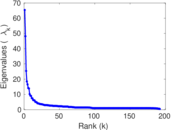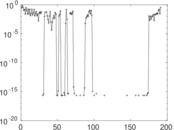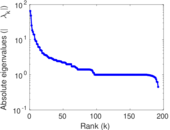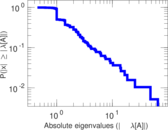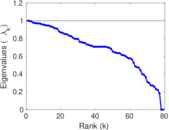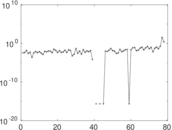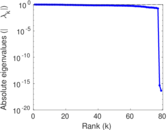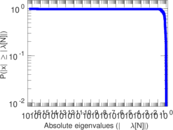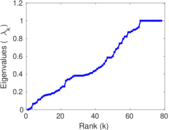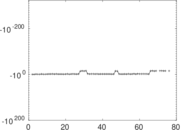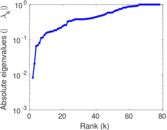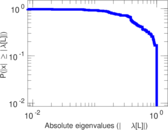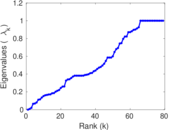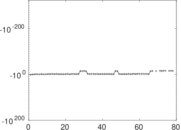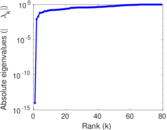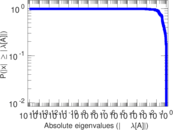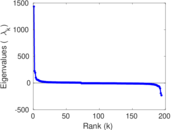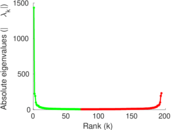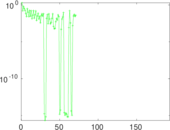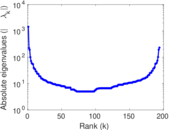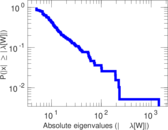# Wikibooks edits (ang)

This is the bipartite edit network of the Old English Wikibooks. It contains users and pages from the Old English Wikibooks, connected by edit events. Each edge represents an edit. The dataset includes the timestamp of each edit.

 Code `bang` Internal name `edit-angwikibooks` Name Wikibooks edits (ang) Data source http://dumps.wikimedia.org/ AvailabilityDataset is available for download Consistency checkDataset passed all tests Category Authorship network Dataset timestamp 2017-10-20 Node meaning User, article Edge meaning Edit Network formatBipartite, undirected Edge typeUnweighted, multiple edges Temporal dataEdges are annotated with timestamps

## Statistics

 Size n = 1,436 Left size n1 = 194 Right size n2 = 1,242 Volume m = 2,480 Unique edge count m̿ = 1,614 Wedge count s = 276,863 Claw count z = 57,104,817 Cross count x = 9,528,405,963 Square count q = 19,518 4-Tour count T4 = 1,270,388 Maximum degree dmax = 1,202 Maximum left degree d1max = 1,202 Maximum right degree d2max = 48 Average degree d = 3.454 04 Average left degree d1 = 12.783 5 Average right degree d2 = 1.996 78 Fill p = 0.006 698 54 Average edge multiplicity m̃ = 1.536 56 Size of LCC N = 1,135 Diameter δ = 16 50-Percentile effective diameter δ0.5 = 3.221 58 90-Percentile effective diameter δ0.9 = 5.391 35 Median distance δM = 4 Mean distance δm = 3.692 91 Gini coefficient G = 0.664 632 Balanced inequality ratio P = 0.244 153 Left balanced inequality ratio P1 = 0.131 048 Right balanced inequality ratio P2 = 0.349 194 Relative edge distribution entropy Her = 0.772 351 Power law exponent γ = 4.856 37 Tail power law exponent γt = 2.121 00 Tail power law exponent with p γ3 = 2.121 00 p-value p = 0.395 000 Left tail power law exponent with p γ3,1 = 1.911 00 Left p-value p1 = 0.631 000 Right tail power law exponent with p γ3,2 = 6.251 00 Right p-value p2 = 0.000 00 Degree assortativity ρ = −0.154 544 Degree assortativity p-value pρ = 4.335 02 × 10−10 Spectral norm α = 65.390 1 Algebraic connectivity a = 0.008 287 00 Spectral separation |λ1[A] / λ2[A]| = 1.357 30 Controllability C = 1,047 Relative controllability Cr = 0.731 656

## Plots

### Fruchterman–Reingold graph drawing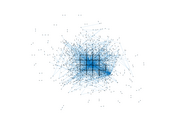### Degree distribution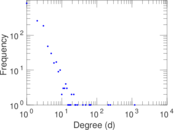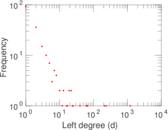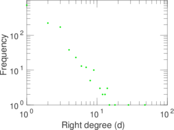### Cumulative degree distribution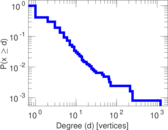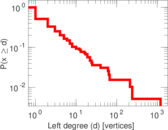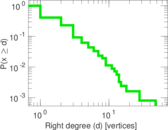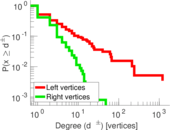### Lorenz curve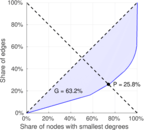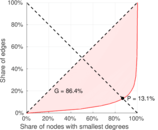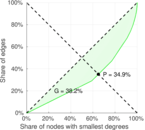### Spectral distribution of the adjacency matrix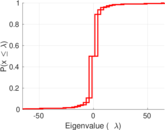### Spectral distribution of the normalized adjacency matrix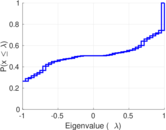### Spectral distribution of the Laplacian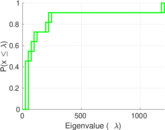### Spectral graph drawing based on the adjacency matrix### Spectral graph drawing based on the Laplacian### Spectral graph drawing based on the normalized adjacency matrix### Degree assortativity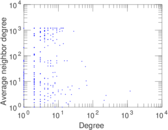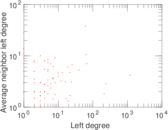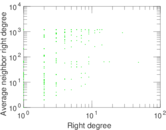### Zipf plot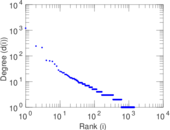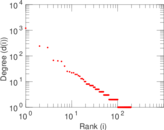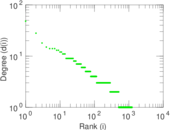### Hop distribution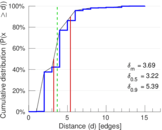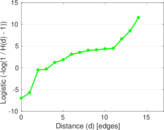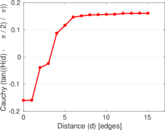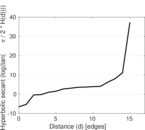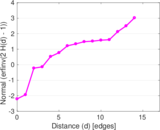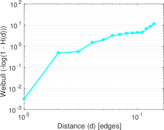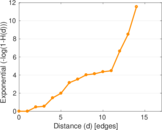### Double Laplacian graph drawing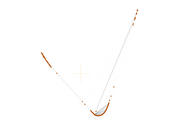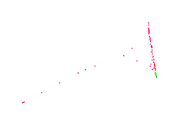### Delaunay graph drawing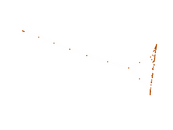### Edge weight/multiplicity distribution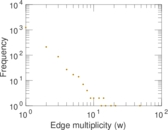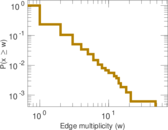### Temporal distribution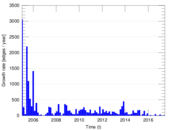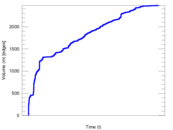### Temporal hop distribution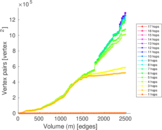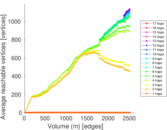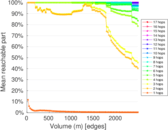### Diameter/density evolution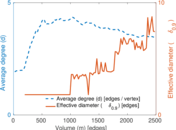### Matrix decompositions plots